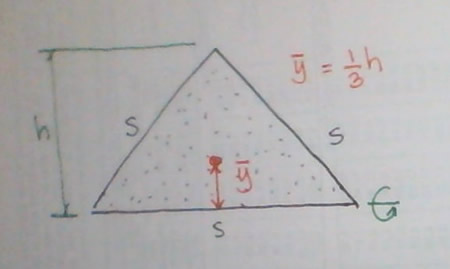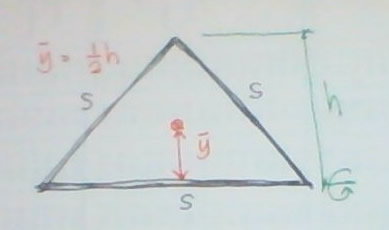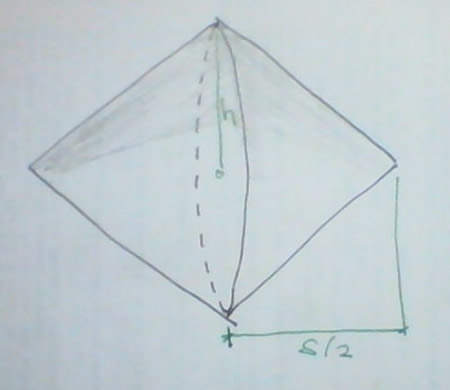# equilateral triangle revolved about one of its sides

2 posts / 0 newequilateral triangle revolved about one of its sides

find the area and volume of the figure developed by an equilateral triangle with sides s if it is revolved about one of its sides.

Jhun VertUse Pappus theorem:
V = volume generated
A = surface area generated
At = generating area
L = generating length of curve
C = distance traveled by the centroid

$h = \sqrt{s^2 - (\frac{1}{2}s)^2} = \frac{\sqrt{3}}{2}s$

$\bar{y} = \frac{1}{3}h = \frac{1}{3}(\frac{\sqrt{3}}{2}s) = \frac{\sqrt{3}}{6}s$   ←   centroid of area

$A_t = \frac{1}{2}sh = \frac{1}{2}s(\frac{\sqrt{3}}{2}s) = \frac{\sqrt{3}}{4}s^2$$V = A_t \times C = A_t \times 2\pi \bar{y}$

$V = \frac{\sqrt{3}}{4}s^2 \times 2\pi (\frac{\sqrt{3}}{6}s)$

$V = \frac{1}{4}\pi s^3$

$L = 2s$   ←   the third side, being the axis of rotation, cannot generate a surface

$\bar{y} = \frac{1}{2}h = \frac{1}{2}(\frac{\sqrt{3}}{2}s) = \frac{\sqrt{3}}{4}s$   ←   centroid of lines$A = L \times C = L \times 2\pi \bar{y}$

$A = 2s \times 2\pi (\frac{\sqrt{3}}{4}s)$

$A = \sqrt{3}\pi s^2$

You can consider the volume generated as a cone, there will be two cones involved with common bases. The radius of each cone is h and the height is s/2.
$V = 2 \times \frac{1}{3}\pi h^2 (\frac{1}{2}s)$

$V = 2 \times \frac{1}{3}\pi (\frac{\sqrt{3}}{2}s)^2 (\frac{1}{2}s)$

$V = \frac{1}{4}\pi s^3$For the surface area generated, it is the lateral area of two cones
$A = 2 \times \pi hs = 2 \times \pi (\frac{\sqrt{3}}{2}s)s$

$A = \sqrt{3}\pi s^2$

Subscribe to MATHalino on